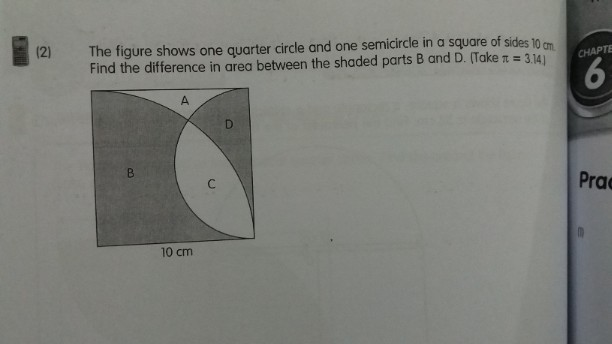# QuestionThis is a Area question.

1. Area of quadrant B C = 1/4 × 10 × 10 × pi = 25 pi

2. Area of semicircle CD = 1/2 × 5 × 5 × pi = 12.5 pi

3. Difference in Area = 25 pi – 12.5 pi = 12.5pi

= 12.5 × 3.14 = 39.25

😊

Note

Difference in Area mean one shape minus the other when they do not overlap totally.

😊😊

0 Replies 0 Likes